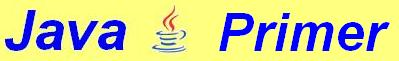2. Drawing in an applet

The paint() method is - as the name suggests - very good at drawing. Let's draw a circle whose centre is the same as the centre of the applet window. The Graphics class has a method to draw an ellipse, which looks like this:

drawOval (x, y, width, height);

This will give us an ellipse (or "oval") whose measures are dependent on the "enclosing rectangle", like this: If you want to draw the rectangle which is shown in the diagram in dotted lines, you can do it with:
g.drawRect (x, y, width, height);
A circle is really an ellipse of equal width and height. Let's draw a circle whose centre is the same as the centre of the applet window. But as you can see, the centre plays no part at all in the method drawOval (x,y,width, height). So we'll have to do a few calculations.

In the diagram below you can see that x and y can be calculated if you know the coordinates of the centre (xm, ym) and the radius (r). So:
x = xm - r
y = ym - r

The applet window used in this tutorial is 320 pixels wide and 240 pixels high. So we can calculate x and y (for a circle with a radius of 100 pixels in the middle of the applet window) as follows:

x = 320/2 - r = 160 - 100 = 60
y = 240/2 - r = 120 - 100 = 20
The following applet draws the circle:
// A circle drawn through the
// centre of the applet window
import java.applet.*;
import java.awt.*;
public class CircleThroughCentre extends Applet {
public void paint (Graphics g)  {
g.drawOval (60, 20, 200, 200);
}

}

If we want to give this circle a different radius, we would have to change all four numbers mentioned after drawOval - whereas it is in fact just one thing that changes: the radius. It would be much better if we make use of variables.

We can store the length (100 pixels) of the radius as follows:

This reserves a piece of memory for storing a whole number - or integer (int), which we call radius. We can also store the width and height of the applet window into variables in this way:

int width = 320, height = 240;

The use of variables makes programs more efficient and a lot easier to adapt. With variables our circle-applet looks like this:

// A circle drawn through the middle of
//    the applet window using variables.
import java.applet.*;
import java.awt.*;
public class
CircleThroughCentre extends Applet {

public void paint (Graphics g) {
}
}

Variables with text: strings
The variables we have seen so far were of the type int (integers, whole numbers).

A very special sort of variables are the so-called strings. They consist of series of characters. They can contain any sort of character, for example:
String name = "Linus Torvalds";
String line = "--------------------------";
String sum = "2 + 3 = 5";
Later in this tutorial you'll see that strings offer interesting possibilities. But you can't calculate with them. Just look at this program code:
String s1 = "12", s2 = "13";
String text = "The sum of " + s1 + " and " + s2;
g.drawString (text + " is " + s1 + s2, 10, 100);
The output (below) maybe somewhat surprising:

 Exercise 2.1 Write an applet which depicts two rectangles of the same size next to each other. The distance between the rectangles and the distance to the right and left side of the window must be equal to the width of each of the rectangles. The distance to the top and the bottom must be equal to the height of the rectangles. Don't calculate the measures with numbers, but with these two variables: int width = 320, height = 240; In this way, you can just change the size of the applet window, and the rectangles will remain correct.
To chapter 3